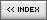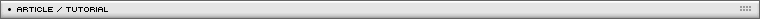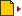Projection Plane Based Real 3D
[ January 03, 2005 ] by Milan Toth (MilGra)
How to simulate 3D animation using plane projections.

All children, from the age of 6 dreams of an own 3D engine. Even I did that. That's why i have written this tutorial, to make those people`s work easier who couldn't succeeded in doing so.

Part 1. The theory

What is it about? We have a point in the 3 dimensional space, and we would like to project it perpendicularly to an arbitrary plane, and we have to determine the projected points position relative to a given point on the plane. We can define a plane in many ways, but in this case the best way is to define it by a normal vector and a base point, so it will be much more easier to move it. In addition, we can at once use the normal vector as the position vector of the line going through the chosen point in the 3D space.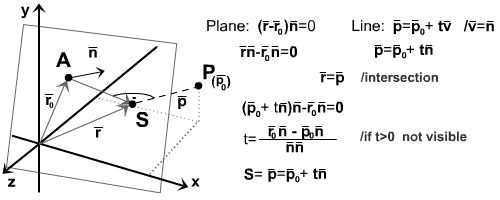Figure 1

We can define the intersection point by using the parametric equations of the line (normal to the plane, going through the point P) and the plane. However, we can't determine a relative position directly yet, because we only know one point related to the plane. We need two more points related to the plane with which we will be able to determine the position of S.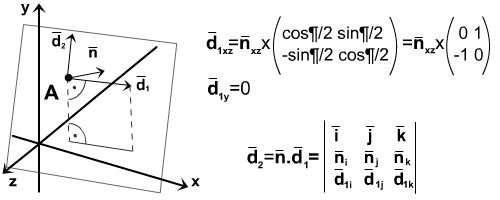Figure 2

The simplest way is to rotate the plane's normal onto the plane to such a position where it is parallel to xz plane thus providing a fix axis. The other vector can be calculated by the vectorial multiplication of the normal and the previously created vector. If we have all this, then we only need to calculate the relative position of S.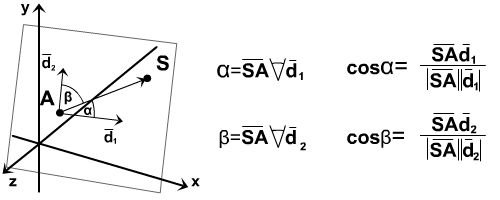Figure 3

We only need to determine the angles between SA vector, and the directional vectors, and we have to change into the planes A origined, 2 dimensional coordinate system.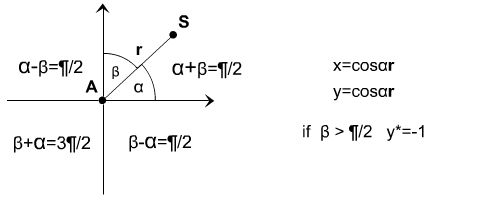Figure 4

Part 2. All this in practice

Everything has been good and easy so far, but how the hell can we implement this? So, it's no easy matter, i suffered for 3 weeks, until i managed to structure the code in a simple and clean form.
We need two classes, the first - and the most important - is the Vector class, since we only use vectors during the projection, and the second is the Plane class, in which we can carry out other, plane-related functions.
But let's see the Vector class first. It must have all the used vector operations.

```//
// Vector Class
//
Vector = function (i, j, k)
{
this.i = i;
this.j = j;
this.k = k;
this.r = Math.sqrt(i*i+j*j+k*k);
};

//
// Standard Summa
//
Vector.prototype.Sum = function (ve)
{
var i=this.i+ve.i;
var j=this.j+ve.j;
var k=this.k+ve.k;
return new Vector(i,j,k);
};

//
// Standard Substraction
//
Vector.prototype.Sub = function (ve)
{
var i=this.i-ve.i;
var j=this.j-ve.j;
var k=this.k-ve.k;
return new Vector(i,j,k);
};

//
// Dot (vectorial) Multiplication of two vectors
// Standard 3x3 Matrix Determinant Calculation
//
Vector.prototype.DotMul = function(ve)
{
var i = this.j*ve.k-this.k*ve.j;
var j = -(this.i*ve.k-this.k*ve.i);
var k = this.i*ve.j-this.j*ve.i;
return new Vector(i, j, k);
};

//
// Scalar Multiplication of two Vectors
//
Vector.prototype.VectMul = function(ve)
{
return this.i*ve.i+this.j*ve.j+this.k*ve.k;
};

//
// Multiplication with a Scalar
//
Vector.prototype.SkalMul = function(skal)
{
var i = this.i*skal;
var j = this.j*skal;
var k = this.k*skal;
return new Vector(i, j, k);
};

//
// Calculating angle between two vectors
//
Vector.prototype.Angle = function(ve)
{
var vmult = this.i*ve.i+this.j*ve.j+this.k*ve.k;
var rmult = this.r*ve.r;
var alpha = Math.acos(Math.round(vmult)/Math.round(rmult));
return alpha;
};
```

After this it will be childs play for our plane to project the desired points.

```//
// Plane Class
// constructor receives two base vectors
//
Plane = function (v1, v2)
{
this.v1 = v1;
this.v2 = v2;
this.Init();
};

//
// Calculating additional vectors described in figure 2
// normal vector, d1 (xpar), d2 (ypar)
//
Plane.prototype.Init = function()
{
this.norm_ve = this.v2.Sub(this.v1);
this.xpar_ve = new Vector(-1*this.norm_ve.k, 0, this.norm_ve.i);
this.ypar_ve = this.norm_ve.DotMul(this.xpar_ve);
};

//
// Calculating intersection of line and plane as shown in figure 1
//
Plane.prototype.CrossPoint = function(pt)
{
var dir_ve = new Vector(pt.x, pt.y, pt.z);
var t = (this.v1.VectMul(this.norm_ve)-dir_ve.VectMul(this.norm_ve))
/(this.norm_ve.VectMul(this.norm_ve));
var dx = t*this.norm_ve.i;
var dy = t*this.norm_ve.j;
var dz = t*this.norm_ve.k;
var dist = Math.sqrt(dx*dx+dy*dy+dz*dz);
if (t>0) dist *= -1; //not visible
return {x:pt.x+dx, y:pt.y+dy, z:pt.z+dz, d:dist};
};

//
// Converting 3D coordinates to the plane`s local system, as shown in figure 3 and figure 4
//
Plane.prototype.Project = function(pt)
{
var a = this.CrossPoint(pt);
var b = this.v1;
var dir_ve = new Vector((a.x-b.i), (a.y-b.j), (a.z-b.k));
var alpha = dir_ve.Angle(this.xpar_ve);
var betha = dir_ve.Angle(this.ypar_ve);
if (dir_ve.r == 0)
{
var xc = 0;
var yc = 0;
}
else
{
var xc = Math.cos(alpha)*dir_ve.r;
var yc = -Math.sin(alpha)*dir_ve.r;
}

if (betha>Math.PI/2) yc *= -1;

// setting perspective distortion
var focal = (focuslength-a.d)/focuslength;
xc *= focal;
yc *= focal;
//if t>0 not visible
var infront = a.d < 0 ? 0 : 1;
if ((xc < 200 && xc > -200) && (yc > -200 && yc < 200)) var onscreen=1; else var onscreen=0;
return {x:xc, y:yc, v:infront*onscreen};
};```

And thats all folks! I've further tuned the final version to make things more spectacular, download it and examine it to your hearts content.